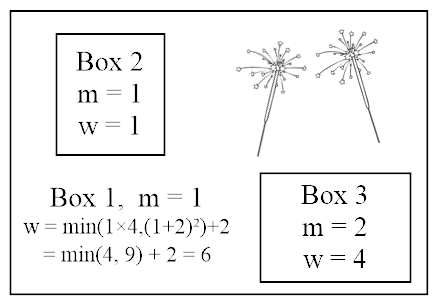## Tenri

Tenri is getting ready to perform a magic show with N (1 ≤ N ≤ 11) magic boxes and S (1 ≤ S ≤ 15) sparklers. Because these boxes are strange and mysterious, each box can hold either zero or two other magic boxes (that are not within any other box yet) and any number of sparklers. Each box has a weight, determined by the formula:

w = min(wi×wj , (m+s)2) + s

where m is the mysteriousness of the box, s is the number of sparklers in it, and wi and wj are the weights of the two boxes within this box. If the box is completely empty, assume a value of infinity for wi×wj. Tenri's magic show requires a single box which contains all the other boxes and sparklers either directly or indirectly. The overall impact for the magic show is the weight of the outermost box. Please determine the maximum impact Tenri's magic show can have.

### Input

The first line of input has 2 integers, N and S, the number of boxes and the number of sparklers Tenri has, respectively. N is guaranteed to be an odd number. Each of the next N lines contains a single positive integer less than or equal to 10, the mysteriousness value of i-th box Tenri has.

### Output

Output the maximum possible impact Tenri's magic show can have.

```3 2
1
1
2
```

```6
```

### Explanation

Tenri can put the second and third of her boxes inside the first box, and then put two sparklers in the first box for an impact value of:

min((min(∞, (1 + 0)2) + 0) × (min(∞, (2 + 0)2) + 0)), (1 + 2)2) + 2 = 6.

A diagram for this setup is as follows:```7 5
1
2
3
4
5
6
7
```

### Sample Output 2

```149
```

Point Value: 20 (partial)
Time Limit: 3.00s
Memory Limit: 64M